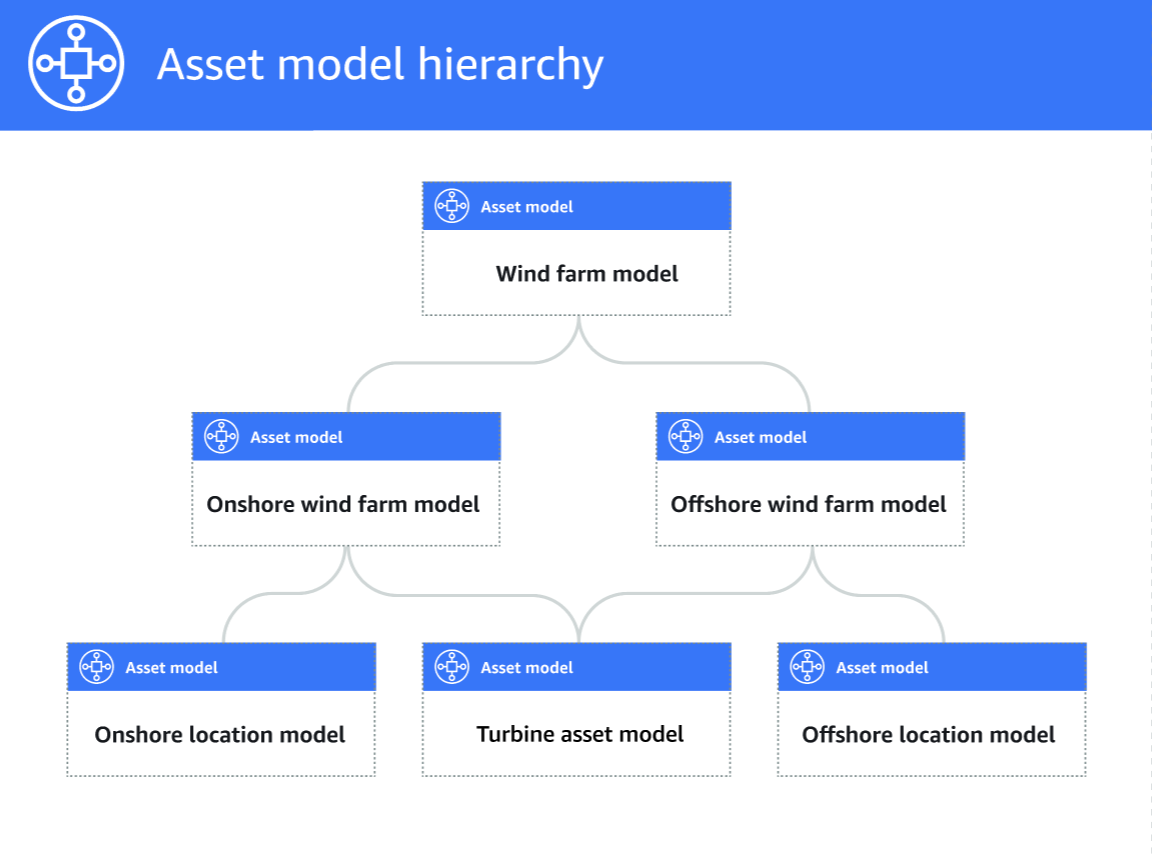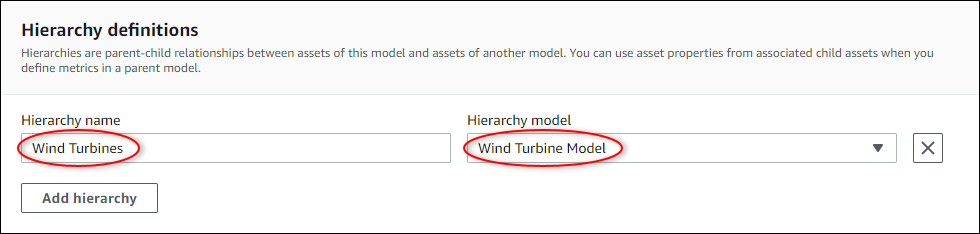Defining relationships between asset models (hierarchies) - Amazon IoT SiteWise
Services or capabilities described in Amazon Web Services documentation might vary by Region. To see the differences applicable to the China Regions, see Getting Started with Amazon Web Services in China (PDF).

# Defining relationships between asset models (hierarchies)

You can define asset model hierarchies to create logical associations between the asset models in your industrial operation. For example, you can define a wind farm composed of onshore and offshore wind farms. An onshore wind farm contains a turbine and onshore location. An offshore wind farm contains a turbine and offshore location.When you associate a child asset model to a parent asset model through a hierarchy, the parent asset model's metrics can input data from the child asset model's metrics. You can use asset model hierarchies and metrics to calculate statistics that provide insight to your operation or a subset of your operation. For more information, see Aggregating data from properties and other assets (metrics).

Each hierarchy defines a relationship between a parent asset model and a child asset model. In a parent asset model, you can define multiple hierarchies to the same child asset model. For example, if you have two different types of wind turbines in your wind farms, where all wind turbines are represented by the same asset model, you can define a hierarchy for each type. Then, you can define metrics in the wind farm model to calculate independent and combined statistics for each type of wind turbine.

A parent asset model can be associated with multiple child asset models. For example, if you have an onshore wind farm and an offshore wind farm that are represented by two different asset models, you can associate these asset models with the same parent wind farm asset model. A child asset model can also be associated with multiple parent asset models. For example, if you have two different types of wind farms, where all wind turbines are represented by the same asset model, you can associate the wind turbine asset model with different wind farm asset models.

###### Note

When you define an asset model hierarchy, the child asset model must be `ACTIVE` or have a previous `ACTIVE` version. For more information, see Asset and model states.

After you define hierarchical asset models and create assets, you can associate the assets to complete the parent-child relationship. For more information, see Creating assets and Associating and disassociating assets.

## Defining asset model hierarchies (console)

When you define a hierarchy for an asset model in the Amazon IoT SiteWise console, you specify the following parameters:

• Hierarchy name – The hierarchy's name, such as `Wind Turbines`.

• Hierarchy model – The child asset model.

###### Example hierarchy definition

The following example demonstrates an asset model hierarchy that represents a wind farm's relationship to wind turbines.## Defining asset hierarchies (CLI)

When you define a hierarchy for an asset model with the Amazon IoT SiteWise API, you specify the following parameters:

• `name` – The hierarchy's name, such as ```Wind Turbines```.

• `childAssetModelId` – The ID of the child asset model for the hierarchy. You can use the ListAssetModels operation to find the ID of an existing asset model.

###### Example hierarchy definition

The following example demonstrates an asset model hierarchy that represents a wind farm's relationship to wind turbines. This object is an example of an AssetModelHierarchy. For more information, see Creating an asset model (CLI).

``````{
...
"assetModelHierarchies": [
{
"name": "Wind Turbines",
"childAssetModelId": "a1b2c3d4-5678-90ab-cdef-11111EXAMPLE"
},
]
}``````